# Match the vapor pressure diagrams with the solute-solvent combinations and explain your answers. a. and b. and c. and d. and### Chemistry: An Atoms First Approach

2nd Edition
Steven S. Zumdahl + 1 other
Publisher: Cengage Learning
ISBN: 9781305079243

#### Solutions

Chapter
Section### Chemistry: An Atoms First Approach

2nd Edition
Steven S. Zumdahl + 1 other
Publisher: Cengage Learning
ISBN: 9781305079243
Chapter 10, Problem 63E
Textbook Problem
22 views

## Match the vapor pressure diagrams with the solute-solvent combinations and explain your answers.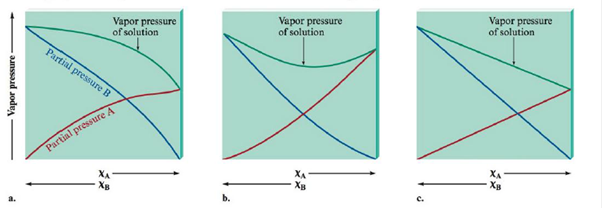a.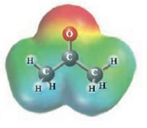and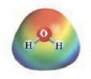b.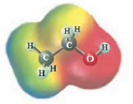andc.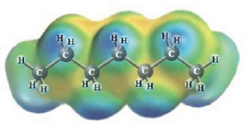and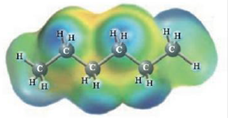d.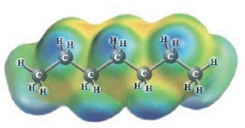and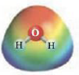(a)

Interpretation Introduction

Interpretation:

The dissolution of the given following solute and solvent has to be explained using Raoult’s law.

Concept Introduction: Concept introduction:

Raoult's law:

The mole fraction of a solute is related to the vapor pressure of the solution thus,

Psolution=P°solventXsolvent......(1)Pisvapor pressureof the solutionsolventpressureof the solventXsolvent mole fraction ofsolvent

### Explanation of Solution

To find the second diagram match with Acetone and Water

The second diagram illustrates negative variation from Raoult's law. This occurs whilst the solute-solvent connections are stronger than the connections in pure solvent and pure solute.

CH3COCH3 and H2O

These two molecules are named Acetone (

(b)

Interpretation Introduction

Interpretation:

The dissolution of the given following solute and solvent has to be explained using Raoult’s law.

Concept Introduction: Concept introduction:

Raoult's law:

The mole fraction of a solute is related to the vapor pressure of the solution thus,

Psolution=P°solventXsolvent......(1)Pisvapor pressureof the solutionsolventpressureof the solventXsolvent mole fraction ofsolvent

(c)

Interpretation Introduction

Interpretation:

The dissolution of the given following solute and solvent has to be explained using Raoult’s law.

Concept Introduction: Concept introduction:

Raoult's law:

The mole fraction of a solute is related to the vapor pressure of the solution thus,

Psolution=P°solventXsolvent......(1)Pisvapor pressureof the solutionsolventpressureof the solventXsolvent mole fraction ofsolvent

(d)

Interpretation Introduction

Interpretation:

The dissolution of the given following solute and solvent has to be explained using Raoult’s law.

Concept Introduction: Concept introduction:

Raoult's law:

The mole fraction of a solute is related to the vapor pressure of the solution thus,

Psolution=P°solventXsolvent......(1)Pisvapor pressureof the solutionsolventpressureof the solventXsolvent mole fraction ofsolvent

### Still sussing out bartleby?

Check out a sample textbook solution.

See a sample solution

#### The Solution to Your Study Problems

Bartleby provides explanations to thousands of textbook problems written by our experts, many with advanced degrees!

Get Started

Find more solutions based on key concepts
The BMI standard is an excellent tool for evaluating obesity in athletes and the elderly. T F

Nutrition: Concepts and Controversies - Standalone book (MindTap Course List)

What is genomics?

Human Heredity: Principles and Issues (MindTap Course List)

Two point charges are on the y axis. A 4.50-C charge is located at y = 1.25 cm, and a -2.24-C charge is located...

Physics for Scientists and Engineers, Technology Update (No access codes included)

What technical innovation made surfing waves of this magnitude possible?

Oceanography: An Invitation To Marine Science, Loose-leaf Versin